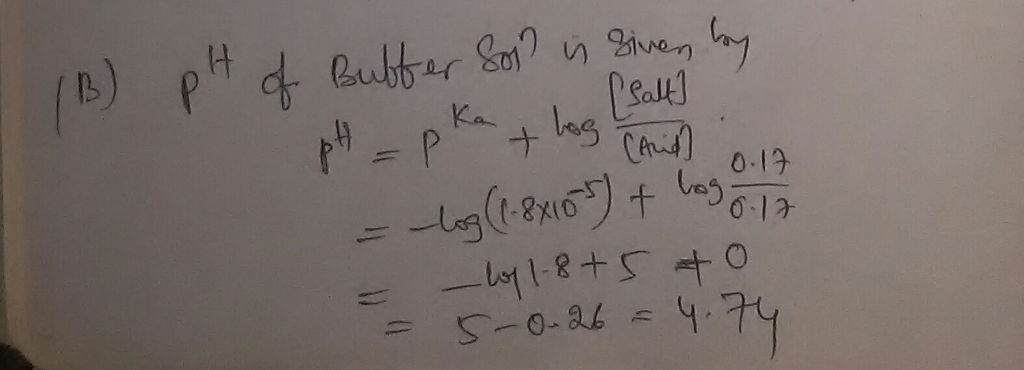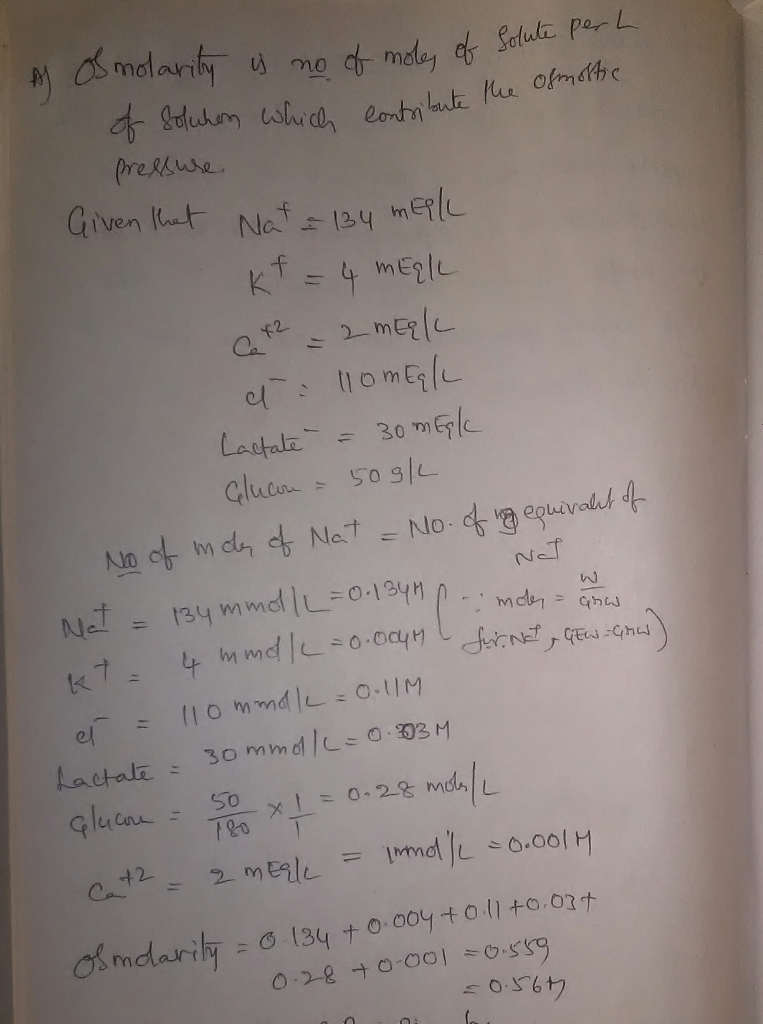# Part A. A lactated Ringer's solution with 5% glucose contains Na+ 134 mEq/L , K+ 4 mEq/L , Ca2+ 2...

Part A. A lactated Ringer's solution with 5% glucose contains Na+ 134 mEq/L , K+ 4 mEq/L , Ca2+ 2 mEq/L , Cl− 110. mEq/L , lactate− 30. mEq/L , and 50. g/L glucose (C6H12O6). What is the osmolarity (Osm) of the solution? Express the osmolarity to two decimal places.

Part B: Acetic acid has a Ka of 1.8×10−5. What is the pH of a buffer solution containing 0.17 M HC2H3O2 (acetic acid) and 0.17 M C2H3O2−? Express your answer using two decimal places.

Part B:

PH of the buffer solution is given by Henderson's equation.

Given that concentration of acid = 0.17M

Concentration of acetate ion = 0.17M##### Add Answer of: Part A. A lactated Ringer's solution with 5% glucose contains Na+ 134 mEq/L , K+ 4 mEq/L , Ca2+ 2...
More Homework Help Questions Additional questions in this topic.

• #### Sodium sulfate is slowly added to a solution containing 0.0500 M Ca2 *(aq) and 0.0360 M Ag (aq). ...

Need Online Homework Help?